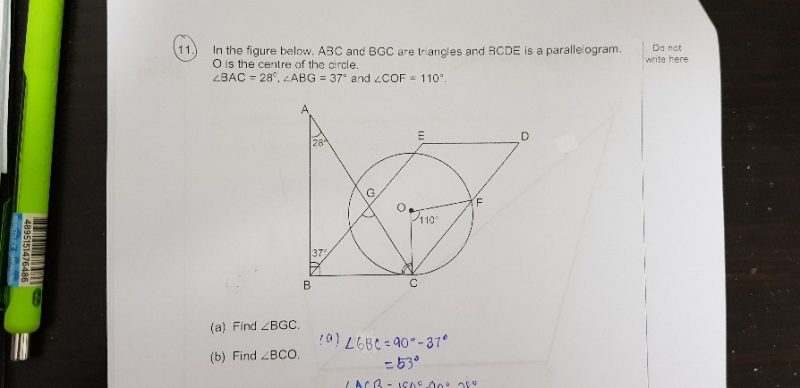# Question1 Answer

# Answer

(a) ∠BGC is the exterior angle of Triangle AGB.

So ∠BGC = ∠BAG  +  ∠ABG  = 28° + 37° = 65°  (Ans)

(b) ∠EBC = 90° -37° = 53°

∠EBC and ∠DCB are interior angles since BE and CD are parallel lines.

so ∠EBC + ∠DCB = 180°

53° + ∠DCB = 180°

∠DCB = 127°

Triangle OCF is isosceles since OC and OF are the radii of the circle.

∠OCF = (180 – 110) ÷ 2 = 35°

∠BCO = ∠DCB – ∠OCF  =  127°  –  35°  = 92°  (Ans)

0 Replies 0 Likes